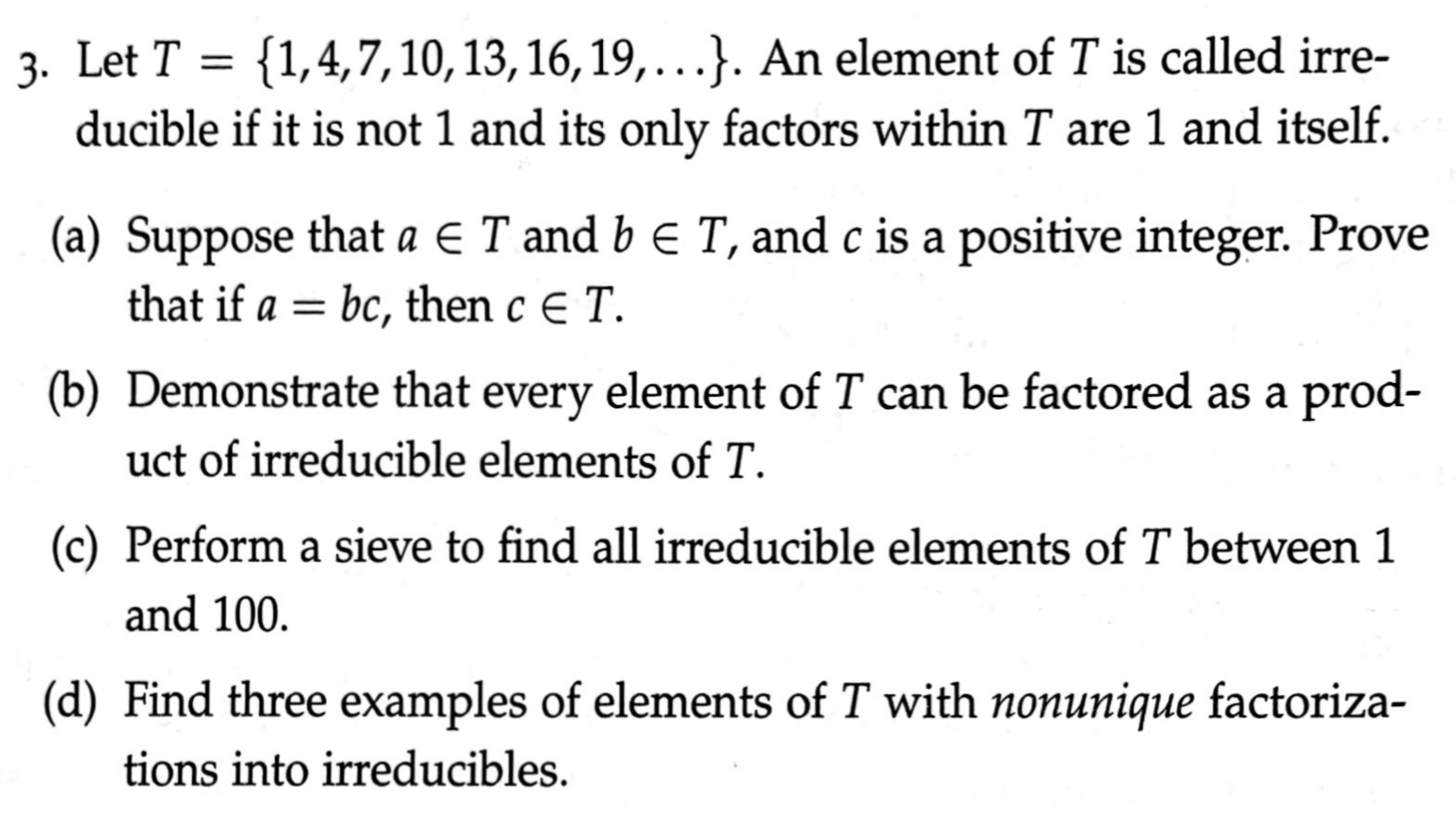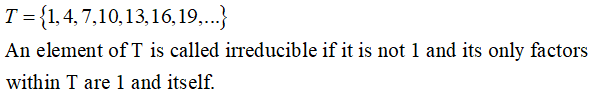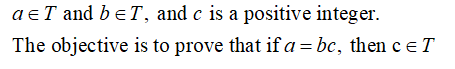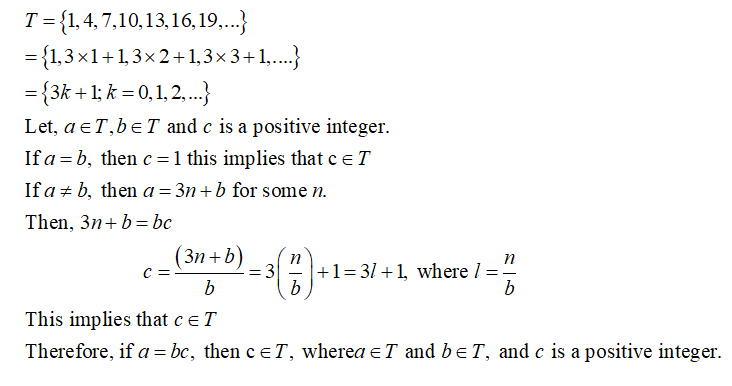# {1,4,7,10, 13, 16, 19,...}. An element of T is called irre-ducible if it is not 1 and its only factors within T are 1 and itself.3. Let T =(a) Suppose that a E T and b e T, and c is a positive integer. Provethat if a = bc, then c E T.(b) Demonstrate that every element of T can be factored as a prod-uct of irreducible elements of T.(c) Perform a sieve to find all irreducible elements of T between 1and 100.(d) Find three examples of elements of T with nonunique factoriza-tions into irreducibles.

Question
45 views

Number Theoryhelp_outlineImage Transcriptionclose{1,4,7,10, 13, 16, 19,...}. An element of T is called irre- ducible if it is not 1 and its only factors within T are 1 and itself. 3. Let T = (a) Suppose that a E T and b e T, and c is a positive integer. Prove that if a = bc, then c E T. (b) Demonstrate that every element of T can be factored as a prod- uct of irreducible elements of T. (c) Perform a sieve to find all irreducible elements of T between 1 and 100. (d) Find three examples of elements of T with nonunique factoriza- tions into irreducibles. fullscreen
check_circle

Step 1

Since we only answer up to 3 sub-parts, we’ll answer the first 3. Please resubmit the question and specify the other subparts (up to 3) you’d like answered.

Given thatStep 2

(a)Suppose thatStep 3

Now,...

### Want to see the full answer?

See Solution

#### Want to see this answer and more?

Solutions are written by subject experts who are available 24/7. Questions are typically answered within 1 hour.*

See Solution
*Response times may vary by subject and question.
Tagged in

### Math